# Abbe-Method

Measuring method (named after Ernst Abbe) used to determine the focal length and the position of the principal planes of a lens singlet or a lens system (=objective) on the optical axis.

How to determine the focal length:
The position of the lens is fixed and the camera (or the screen ) is moved depending on the object position, that you get a focused image (in the image center). Different object positions result in different camera- or screen distancesHow to determine the focal length of an objective (= (= lens system)):
The Position of a lens (and the lens singlets in it) are fixed and an arbitrary Point O on the optical axis is marked as reference point, for example the center of the lens or the center of the first lens element).
Now we measure the distance x from the reference point to the object, the distance x’ to the image and the image size B.
You get a list of Magnifications,
and equations from refererence Point to objectand reference point to image:Where h und h’ are the distances from object side resp. image side principal planw to the reference point.

# Abbe-number

(also known as v-number)
a measure of the materials dispersion (=variation of refractive index with wavelength),
with high values of V indicating low dispersion (low chromatic aberration).

The value Vd is given bywhich defines the Abbe number with respect to the yellow Fraunhofer-Line d (or D3) helium line at 587.5618 nm wavelength.

It can also be defined using the green mercury E-line at 546.073 nm:where F’ and C’ are the blue and red cadmium lines at 480.0 nm and 643.8 nm, respectively.

# Abbe’s Invariant

In paraxial optics each single refracting surface satisfies the Abbe’s Invariant Q in the paraxial Area, that relates the front focal distance s of an axial object point with the back focal distance s’ of it’s conjugated point behind the surface# ABCD Matrix

ABCD Matrixesare used in paraxial optical design.

It’s only allowed to use ABCD Matrices in the paraxial range, withThe angles are measured in radians!

A beam is described by a distance r from the optical axis and a offset angle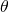from the optical axis
An ABCD Matrix that describes the optical element is formed
The ABCD Matrix is multiplied by the input vector
The result is an output vector that describes the output beam with a new distance from the optical axis and a new angle off the optical axisis a short for for the equation systemwithwith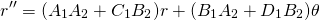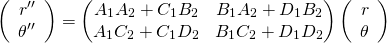Examples of ABCD matrices for simple optical elements :

Propagation in free space or in a medium of constant refractive index :Where d = reduced distance= thickness / refraction index
Propagation through a series i=1..k of planparallel media with constant refraction indices: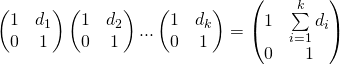Where d_i = reduced distance_i= thickness_i / refraction index_i

Refraction at a flat surface:Where:= initial refractive index= final refractive index
Refraction at a curved surface:Where:
R = radius of curvature,for a convex surface (centre of curvature after interface)= initial refractive index= final refractive index
Reflection vrom a flat mirror:Identity matrix
Reflection from a curved mirror:Where:effective radius of curvature in tangential plane (horizontal direction)effective radius of curvature in the sagittal plane (vertical direction)
Withfor convex mirrors (centre of curvature after interface)
Refraction at a thin lens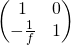Where:
f = focal length of the lens, wherefor convex/positive/converging lenses. Valid if if and only if the focal length is much bigger than the thickness of the lens
Refraction at a thick lensWhere:= refractive index outside of the lens.= refractive index of the lens itself (inside the lens).= Radius of curvature of First surface.= Radius of curvature of Second surface.
t = center thickness of lens.

# aberration

deviation from perfection of an optical system.

Even a perfectly produced optical system has aberrations. Production does produce additional aberrations.

# Aperture angle

Angle that the lens can see in the direction of a given sensor measure.

The angles in horizontal, diagonal and vertical directions differ.
Please distinguish between the max. possible aperture angle and the actual aperture angle.
Actual aperture angles are influenced by the length of used extension rings,and even focus distances (because they are mostly achieved by simulating extension rings) max possible aperture angles aren’t
Changing the sensor size changes the actual aperture angle, max possible aperture angles aren’t
Datasheets of lenses usually show aperture angles for a given sensor size! Changing the sensor size changes the angles!
For lenses without distortion a lens that has the diagonal of the sensor as focal length has 53.1 degrees
A (no distortion) 6mm lens on a 1/3″ sensor,a 8mm lens on a 1/2″ sensor and a 16mm lens on a 1″ sensor have the same diagonal aperture angle of 53.1 degrees

# aperture value

Number which characterizes the luminous sensitivity of a lens. Another term for aperture value is F-number.The smaller the number, the more light a lens can collect, the brighter the image, the smaller the depth of field.

The larger the number, the darker the image, but in generally the greater depth of field. At the same time, we generally lose resolution, see Rayleigh Criterion.

The f-number is the ratio of the focal length divided by the apparent size of the aperture (= entrance pupil diameter).

An f = 50mm lens with an F-number of F2.0  has to have least one front lens ofdiameter.
A lens with a focal length of f = 75, diameter 30mm and an F-number of F1.0  can’t exist!

The inverse of the square of the f-number is a measure for the image brightness of a lens.

The image through a F4.0 lens only has a quarter of the brightness of an F2.0 lens, sincefour times smaller than.
The image through a F5.6 lens has about twice the brightness of an image through an F8 lens, sinceand